Outline of stemNRICH

Age 14 to 18 Challenge Level:

stemNRICH consists of 4 applications nodes and 2 mathematics nodes, as shown in the table and diagram below. Although the problems are located in discrete nodes, there is some natural overlap between the various areas, particularly chemistry/biology and physics/engineering.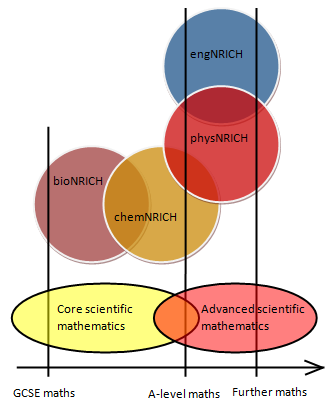At a basic level, all problems refer to GCSE level concepts in science and mathematics, although concepts from A-level mathematics and science will be needed in places.

Most problems come equipped with a solution, hint and teachers' notes pages. There is a wide range of activity-types, so you are sure to practise a variety of mathematical skills.

Core Maths

GCSE mathematics concepts

chemNRICH

GCSE chemistry needed
A-level chemistry needed in places

bioNRICH

GCSE biology needed
A-level biology needed in places
• Numbers
• Algebra, equations and graphs
• Curve fitting and sketching
• Approximations and estimations
• Powers and roots
• Data handling
• Trigonometry
• Units
• Numerical methods, spreadsheets and IT
• General mathematics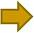• Proportional reasoning
• Dilutions
• Logarithms and pH
• Measurement and estimation
• Reaction kinetics
• Gases
• Bonds and crystals
• Enthalpy
• Moles and molarity
• Articles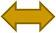• Basic maths in biology
• Measurement and estimation
• Graphs and equations
• Statistics in biology
• Moles and molarity
• Dilutions
• DNA and Genetics
• Shapes
• Articles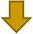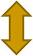A-level mathematics needed
A-level further mathematics needed in placesphysNRICH

A-level physics and mathematics needed
A-level further mathematics needed in places

engNRICH

A-level physics and mathematics needed
A-level further mathematics needed in places
• Rates of change
• Curves
• Calculus
• Differential equations
• Series and expansions
• Powers, roots and logarithms
• Probability and distributions
• Statistics
• Trigonometry
• Geometry and symmetry
• Complex numbers
• Vectors
• Vectors and matrices
• Numerical methods
• General mathematics• General
• Dynamics
• Gravitation
• Units in physics
• Power, work and energy in physics
• Atoms and particles
• Electricity
• Fluids
• Gas laws and thermodynamics
• Waves and oscillations